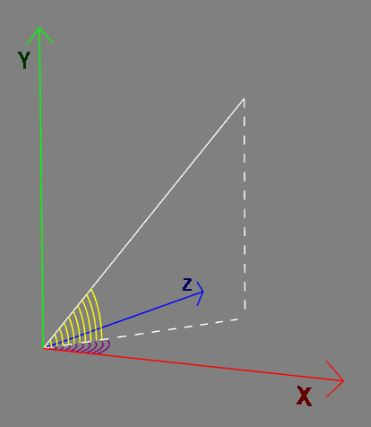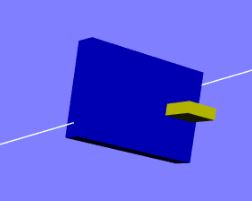# Design for Create Track

This page explains the design behind the 'createTrack' function which you use to build tracks that provide data for a carriage to follow. A track is built for an array of points (Vector3s) which is split into sections. Each section uses the designated lean, the rotation of the carriage about the track direction and turn, the rotation of the carriage about its local Y axis, of the carriage following the track to produce arrays of matrices for each point on the track path. The direction of a rail is given by the tilt (-π/2 to π/2) of the rail about the z axis and its swivel ( to to 2π) about the y axis.

## Rotation Matrices

Matrices are used since they are the fundamental operations needed to combine rotations in a given order. To produce the final rotation of a carriage at any point all the following rotations: tilt; swivel; lean; turn; need to be combined.<------track tilt (yellow angle) and swivel (purple angle)<------carriage lean and turn

## Obtaining the Track Data

The track data produce by `createTrack` are the arrays

• directions: directions of travel;
• rotations: a combination of the lean and turn rotations;
• carriageRotations: lean rotations;
• passengerRotations: turn rotations.

The parameters needed for each section are

• start index;

then the options

• lean;
• leanTwists;
• leanWaves;
• leanWaveAngle;
• turn;
• turnTwists;
• turnWaves;
• turnWaveAngle.

are used with

``````var section = new sectionData(startAt, options)
``````

The code for which is

``````var sectionData = function (startAt, options) {
this.start = startAt;
this.options = options;
}
``````

This data for each section is passed as an array to 'createTrack' along with the points for the track as

``````var createTrack = function(points, sections) {
``````

Internally 'createTrack' uses a 'createSection' function to construct the data to return.

## Section Build

The 'createSection' takes three parameters, points, the full points array for the track, the startSection which contains the information to be used in creating the section and endSection which just provides the lean and turn values at the end of the current section.

The term rail is used to indicate a segment of track from points[i] to points[i + 1]

``````function createSection(points, startSection, endSection) {
/***** Calculate the number of rails for the section *****/
var railsFrom = startSection.start;  //index of start of section
var railsTo = endSection.start; //index of end of section
if(endSection.start === 0) {
railsTo = points.length;
}

var nbRails = railsTo - railsFrom;

/***** Set the defaults for the section *****/
var initialLean = (startSection.options.lean === void 0) ? 0 : startSection.options.lean; //lean of carriage about direction axis at start, a phi variable
var initialTurn = (startSection.options.turn === void 0) ? 0 : startSection.options.turn; // turn of carriage around upright at start, a theta variable
var leanTwists  = (startSection.options.leanTwists === void 0) ? 0 : startSection.options.leanTwists; //number of  lean twists (+ve counter clockwise, -ve clockwise)
var leanWaves  = (startSection.options.leanWaves === void 0) ? 0 : startSection.options.leanWaves; //number of lean waves
var leanWaveAngle  = (startSection.options.leanWaveAngle === void 0) ? 0 : startSection.options.leanWaveAngle; //angle for lean wave
var turnTwists  = (startSection.options.turnTwists === void 0) ? 0 : startSection.options.turnTwists; //number of  turn twists (+ve counter clockwise, -ve clockwise)
var turnWaves  = (startSection.options.turnWaves === void 0) ? 0 : startSection.options.turnWaves; //number of turn waves
var turnWaveAngle  = (startSection.options.turnWaveAngle === void 0) ? 0 : startSection.options.turnWaveAngle; //angle for turn wave

var finalLean = (endSection.options.lean === void 0) ? 0 : endSection.options.lean;
var finalTurn = (endSection.options.turn === void 0) ? 0 : endSection.options.turn;

/***** Check if both waves and  twists are used and ignore twists when so  *****/
//lean waves supersede lean twists unless leanWaveAngle = 0
if (leanWaves > 0 && Math.abs(leanTwists) > 0) {
if (leanWaveAngle == 0) {
leanWaves = 0;
}
else {
leanTwists = 0;
}
}

//turn waves supersede turn twists unless turnWaveAngle = 0
if (turnWaves > 0 && Math.abs(turnTwists) > 0) {
if (turnWaveAngle == 0) {
turnWaves = 0;
}
else {
turnTwists = 0;
}
}

/***** Initiate the variables and their types *****/
//rail transformation matrices for swivel and tilt rotations
var rotationMatrixY = BABYLON.Matrix.Identity();
var rotationMatrixZ = BABYLON.Matrix.Identity();
var rotationMatrix = BABYLON.Matrix.Identity();

var m = BABYLON.Matrix.Identity();
var initialRailDirection = BABYLON.Axis.X;
var initialUprightDirection = BABYLON.Axis.Y;
var initialLevelDirection = BABYLON.Axis.Z;
var railDirection = BABYLON.Vector3.Zero();
var uprightDirection = BABYLON.Vector3.Zero();  //contains vector normal to rail direction after tilt
var levelDirection = BABYLON.Vector3.Zero(); //contains vector normal to rail direction after swivel
var leanDirection = BABYLON.Vector3.Zero(); // contains vector normal to rail direction after tilt, swivel and lean
var turnDirection = BABYLON.Vector3.Zero();
var carriageNormal = BABYLON.Vector3.Zero();
var rotationMatrixLean = BABYLON.Matrix.Identity();
var rotationMatrixTurn = BABYLON.Matrix.Identity();
var rotationMatrixPassenger = BABYLON.Matrix.Identity();
var initialPosition = BABYLON.Vector3.Zero();
var rotation = BABYLON.Matrix.Identity(); //Overall rotation after tilt, swivel, lean and turn

var tilt  = 0; //tilt angle of rail after rotation about (0, 0, 1)
var swivel = 0 //swivel angle of rail after rotation around (0, 1, 0)

var railCount = 0; // determines how many rails along a section, used to control wave values

//Set calculated values for variables
var phi = initialLean;
var theta = initialTurn;

var gradLean = (finalLean - initialLean) / (nbRails - 1); // lean gradient
var gradTurn = (finalTurn - initialTurn) / (nbRails - 1); // turn gradient

var deltaPhi = (finalLean  + 2 * leanTwists * Math.PI - initialLean) / (nbRails); //increase in phi per rail for lean twist
var deltaTheta = (finalTurn  + 2 * turnTwists * Math.PI - initialTurn) / (nbRails); //increase in theta per rail for lean twist

/***** Loop over rails in section*****/

for (var i = railsFrom; i < railsTo; i++) {
points[(i + 1) % points.length].subtractToRef(points[i], railDirection);  //direction of each rail
railDirection.normalize();
swivel = -Math.atan2(railDirection.z, railDirection.x);  //swivel angle rail makes in xz plane
tilt = Math.atan2(Math.abs(railDirection.y), Math.abs(railDirection.x)); // tilt angle rail makes in first quadrant of xy plane
tilt *= Math.sign(railDirection.y); //tilt angle of rail in xy plane from -pi/2 to pi/2
BABYLON.Matrix.RotationAxisToRef(BABYLON.Axis.Y, swivel, rotationMatrixY); //swivel angle to matrix rotation
BABYLON.Matrix.RotationAxisToRef(BABYLON.Axis.Z, tilt, rotationMatrixZ); //tilt angle to matrix rotation
rotationMatrixZ.multiplyToRef(rotationMatrixY, rotationMatrix); // form combined swivel and tilt matrix
BABYLON.Vector3.TransformNormalToRef(initialUprightDirection, rotationMatrix, uprightDirection); //swivel, tilt act on carriage upright
BABYLON.Vector3.TransformNormalToRef(initialLevelDirection, rotationMatrix, levelDirection); //swivel, tilt act on carriage level
uprightDirection.normalize();
levelDirection.normalize();

if (leanWaves > 0) {
phi = initialLean + railCount * gradLean + leanWaveAngle * Math.sin(railCount * leanWaves * Math.PI / (nbRails - 1));
}
else {
phi += deltaPhi;
}
if (turnWaves > 0) {
theta = initialTurn + railCount * gradTurn + turnWaveAngle * Math.sin(railCount * turnWaves * Math.PI / (nbRails - 1));
}
else {
theta += deltaTheta;
}
railCount++;
BABYLON.Matrix.RotationAxisToRef(railDirection, phi, rotationMatrixLean); // lean matrix
BABYLON.Vector3.TransformNormalToRef(uprightDirection, rotationMatrixLean, carriageNormal); // lean applied to upright
BABYLON.Matrix.RotationAxisToRef(carriageNormal, theta, rotationMatrixTurn); // turn applied to upright after lean

BABYLON.Matrix.RotationAxisToRef(initialUprightDirection, theta, rotationMatrixPassenger); //just turn applied to upright
passengerRotations.push(rotationMatrixPassenger.clone());

rotationMatrix.multiplyToRef(rotationMatrixLean, rotation); //rotation from swivel, tilt, and lean only
carriageRotations.push(rotation.clone());
rotation.multiplyToRef(rotationMatrixTurn, rotation); //complete rotation from swivel, tilt, lean and turn
rotations.push(rotation.clone())

directions.push(railDirection.clone());
}
}
``````

## Create Track from Sections

The createTrack function requires the points for the path of the track and an array of sections. Checking is carried out on the order of sections.

``````var createTrack = function(points, sections) {
//Data arrays
var directions = [];
var rotations = [];
var carriageRotations = [];
var passengerRotations = [];

var nbSections = sections.length;

var looped = (sections[nbSections - 1].start === 0);
for(var i = 1; i < nbSections - looped; i++) {
if (sections[i - 1].start > sections[i].start) {
console.log("sections not in order");
return;
}
}
if (0 < sections[nbSections - 1].start && sections[nbSections - 2].start > sections[nbSections - 1].start) {
console.log("last section not in order");
return;
}
var section = sections;
if (section.start > 0) {
startSection = new sectionData(0, {});
sections.unshift(startSection);
nbSections = sections.length;
}

if (0 < sections[nbSections - 1].start && sections[nbSections - 1].start < points.length - 1) { //assume need to close loop
var endSection = new sectionData(0, sections.options);
sections.push(endSection);
}

//Store track data per section
for (var i = 0; i < sections.length - 1; i++) {
createSection(points, sections[i], sections[i + 1]);
}

return {directions: directions, rotations:rotations, carriageRotations: carriageRotations, passengerRotations: passengerRotations}

/****** createSection function goes here *******/

}
``````